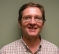## FFT and IFFT scaling factor in OFDMStarted by 6 years ago1 replylatest reply 6 years ago935 views

Hello Everyone

I had a question about normalizing OFDM transmit power after IFFT across each subcarrier. I have simulated OFDM with BPSK and according to different website I have used NFFT/sqrt(Nsubcarrier_used)*ifft(x) where x is the modulated signal and NFFT is the size of ifft and Nsubcarrier_used is total number of subcarrier that is used. This gives me the transmit power of 11 across each subcarrier.

My question is: How to know the scaling factor, such as NFFT/sqrt(Nsubcarrier_used), of different modulation schemes?

[ - ]The way I look at it is in terms of power.  Power is proportional to V^2.  So for an N point FFT/IFFT the sum over N points of I^2 + Q^2 should be the same for both the time domain and the frequency domain.  Find your scaling factor from that.

Most FFT schemes have a scaling schedule where at each stage there is the option to shift the intermediate product by a bit or two.  That is usually a balance between clipping and using the available dynamic range (noise floor).  Then for equal power the final output needs to be shifted a few bits.

Scaling for different constellations is separate.  Usually you want to set the scale factor so that each constellation has the same average power.  That is available as a neat set of numbers in several standards.

The Docsis 3.1 Physical Layer Spec. has BPSK,QPSK, and up to 16K QAM and is available at:

For other modulation schemes it can be calculated from the average power of all the points in the constellation.

Have fun,

Mark Napier